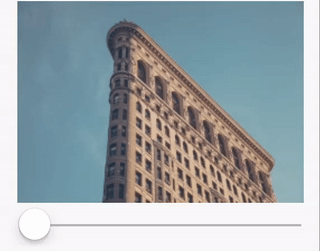# Blur (2-Pass)

``````<Blur width={256} height={180} factor={factor}>
http://i.imgur.com/3On9QEu.jpg
</Blur>
``````## Implementation

The classical example where you need multiple pass is Blur. Implementing an efficient and realist Blur is tricky, one simple and fast way is to implement it with 2-Pass (on X and on Y). To do that, we first define a one dimensional Blur (`Blur1D`) and stack it into these 2 passes.

``````const Blur1D = GL.createComponent(
({ width, height, direction, children }) =>
<GL.Node
width={width}
height={height}
uniforms={{
direction,
resolution: [ width, height ]
}}>
<GL.Uniform name="t">{children}</GL.Uniform>
</GL.Node>
, {
displayName: "Blur1D"
});
``````

And then, we create a Blur that composes Blur1D two times:

``````const Blur = GL.createComponent(({ width, height, factor, children }) =>
<Blur1D width={width} height={height} direction={[ factor, 0 ]}>
<Blur1D width={width} height={height} direction={[ 0, factor ]}>
{children}
</Blur1D>
</Blur1D>
, {
displayName: "Blur"
});
``````

In this simple example, `Blur` has 2 passes: one on X dimension, then one on Y dimension.

### 4-pass

(Used in Examples/Blur)

``````const Blur = GL.createComponent(({ width, height, factor, children }) => {
const sharedProps = { width, height };
return (
<Blur1D {...sharedProps} direction={[ factor, 0 ]}>
<Blur1D {...sharedProps} direction={[ 0, factor ]}>
<Blur1D {...sharedProps} direction={[ -factor/Math.sqrt(2), factor/Math.sqrt(2) ]}>
<Blur1D {...sharedProps} direction={[ factor/Math.sqrt(2), factor/Math.sqrt(2) ]}>
{children}
</Blur1D>
</Blur1D>
</Blur1D>
</Blur1D>
);
});
``````

### N-pass

(Used in Examples/VideoBlur)

``````const GL = require("gl-react");
const React = require("react");
const {
PropTypes
} = React;
const Blur1D = require("./Blur1D");

const NORM = Math.sqrt(2)/2;

function directionForPass (p, factor, total) {
const f = factor * p / total;
switch (p%4) {
case 0: return [f,0];
case 1: return [0,f];
case 2: return [f*NORM,f*NORM];
case 3: return [f*NORM,-f*NORM];
}
return p%2 ? [f,0] : [0,f];
}

module.exports = GL.createComponent(
({ width, height, factor, children, passes, ...rest }) => {
const rec = p => p <= 0 ? children :
<Blur1D {...rest} width={width} height={height} direction={directionForPass(p, factor, passes)}>
{rec(p-1)}
</Blur1D>;
return rec(passes);
},
{
displayName: "Blur",
defaultProps: {
passes: 2
},
propTypes: {
width: PropTypes.number,
height: PropTypes.number,
factor: PropTypes.number.isRequired,
children: PropTypes.any.isRequired,
passes: PropTypes.number
}
});
``````

Usages:

• Small blur:
``````<Blur factor={0.5} passes={2} width={w} height={h}>{any}</Blur>
``````
• Medium blur:
``````<Blur factor={2} passes={4} width={w} height={h}>{any}</Blur>
``````
• Powerful blur:
``````<Blur factor={20} passes={6} width={w} height={h}>{any}</Blur>
``````